# Joules to Newtons

hi guys - this is not a homework problem. i'm way too old to be in school - i'm actually trying to solve a "real world" problem and i'm just a dumb computer scientist pretending to be a mechanical engineer. i'm working on designing a frame for a vehicle and i'm using a couple of different software packages. one of them tells about joules of energy that my frame could get hit by, and the other tells me how resistant my frame by simulating newtons of force on it.

i'd like to understand the equivalence of these two things, so i can sync up my designed between the two software systems.

here's the picture of my question - any help would be very much appreciated.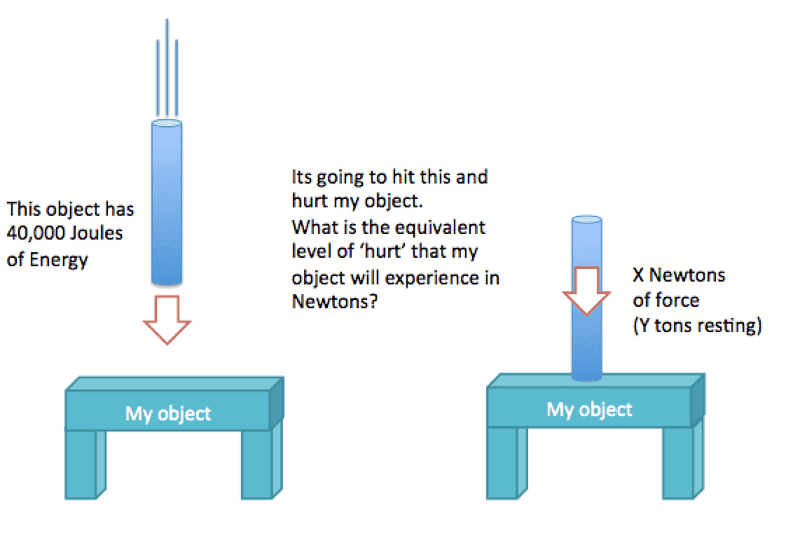I had a similar issue a while back (check my profile if you wish).

Your problem is quite complex, because the forces experienced will depend on the materials involved. The impulse will spread through both "this object" and "my object". This could translate into something like a mean deceleration for "thisy object", and then you have F=m*a. If "this object" is made from rubber, the deceleration will be slower, and thus the forces on "my object" will be lower. Of course, if it's rubber, then a lot of those joules will get lost comrpessing the rubber, but I think you get where I'm going.

I'm sorry I can't be more specific than this, I'm not well versed in these mechanics. Good luck.

jack action
Gold Member
A simplified way of converting one to another is to model your frame as a spring. This assumes that the frame will deform under the hit and go back to its original shape once the force is removed (no permanent deformation). It also assumes the hitting object doesn't deform. Therefore:
$$E = \frac{1}{2}k_f x^2$$
$$F = k_f x$$
Where:
$E$ is the energy of the hitting object;
$k_f$ is the equivalent spring stiffness of the frame;
$x$ is the amount of deformation of the frame;
$F$ is the force produced by the hitting object.

Rearranging to eliminate $x$:
$$F = \sqrt{2k_f E}$$
Now you still have to evaluate $k_f$, which depends on the geometry and type of material, which can be a tedious task.

If you want to add the stiffness of the hitting object into the equation, then:
$$F = \sqrt{2kE}$$
$$k = \frac{k_f k_h}{k_f + k_h}$$
Where:
$k$ is the equivalent spring stiffness of the combined frame and hitting object;
$k_h$ is the equivalent spring stiffness of the hitting object.

And this is a very simplified version to the solution for your problem.

someone suggested something like this - does this sound right to you guys?

The force exerted by the Object on the Rod to bring it to rest is equal to the rate of change of momentum (Newton II):
F = dp/dt

The momentum is:
p = sqrt(2mE)

where E is the energy (assuming non-relativistic energies). By Newton III, this force is equal to the force by the Rod on the Object (how much hurt the Rod will do to the Object).

So basically you need to know how long it takes the Rod to come to rest after contacting the Object (it won't be instant) and the average force will just be:
<F> = sqrt(2mE)/t

where t is the time to come to rest.

jack action
Gold Member
This could work, but estimating t will be a lot harder than k. Actually, the time t will depend on k.

You could just as "easily" do F = E/x (which is based on the definition of work, dE = Fdx) where x is the displacement of the rod between the time the rod comes into contact with the object and the time the rod comes to a stop.

Strength can also vary depending how fast the object is going (for given amount of energy) because some materials are not as strong when subjected to shock loads than when an identical force is applied more gradually.

•Nidum
jack action
Gold Member
To estimate $k$, here is an example for your «My Object».

The 2 vertical rods can be model as a spring with the following spring constant:
$$k_{vr} = \frac{E_mA}{L}$$
Where:
$k_{vr}$ is the spring stiffness of one vertical rod;
$E_m$ is the Young's modulus of the material;
$A$ is the cross section area of the rod;
$L$ is the length of the rod.

For the horizontal beam, if a force $P$ hit the beam in the middle, the deflection $x$ at that point will be $\frac{PL^3}{48E_mI}$ or:
$$P = k_{hb}x$$
$$P = k_{hb}\frac{PL^3}{48E_mI}$$
hence:
$$k_{hb} = \frac{48E_mI}{L^3}$$
Where:
$k_{hb}$ is the spring stiffness of one horizontal beam;
$I$ is the second moment of area of the beam's cross-section.

The equivalent stiffness of two springs in parallel or in series are:

In parallel:
$$k_{eq} = k_1 + k_2$$
In series:
$$k_{eq} = \frac{k_1 k_2}{k_1 + k_2}$$
So, two vertical rods in parallel ($k_{2vr} = k_{vr} + k_{vr}$) are in series with on horizontal beam, hence the stiffness of your frame, $k_f$, is:
$$k_f = \frac{k_{2vr} k_{hb}}{k_{2vr} + k_{hb}}$$
From my previous post:
$$F = \sqrt{2k_f E}$$
or, if you want to also model the hitting object as a spring ($k_h = \frac{E_mA}{L}$):
$$F = \sqrt{2k E}$$
Where:
$$k = \frac{k_f k_h}{k_f + k_h}$$
As the geometry of your frame gets more complex, so does the finding of $k$. But you can often find the «weak spot» which is where you have the lowest spring stiffness and model all other springs $i$ as perfectly stiff rod (i.e. $k_i = \infty$).

And this is the simplest approximation I can think of.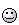When I started this post, I wanted to show you how «easy» it was to estimate $k$, but as I read back this post, I see how many concepts you have to grasp to fully understand what I've just explained and I hope you followed everything.

•Diwakar
JBA
Gold Member
prefech,

What has been given so far is an excellent explanation of the interaction, and complexities, of defection vs energy absorption of two impacting bodies.
With that already addressed, I would like to discuss specifically the engineering elements of your problem; but, first, I need to understand better the basics and givens of the problem.
First, what is your definition of "hurting" your frame, i.e. exceeding the allowable stress limits of the frame members or actually deforming some part of the frame?
Second, are you trying to determine whether the frame will be damaged at some predetermined Impact Energy; or, are you trying to determine the maximum Impact Energy that will cause damage to the frame?

Additionally, do you have an available FEA (finite element analysis) program for determining the load vs stress and deflections of your frame assembly?

prefech,
First, what is your definition of "hurting" your frame, i.e. exceeding the allowable stress limits of the frame members or actually deforming some part of the frame?
Second, are you trying to determine whether the frame will be damaged at some predetermined Impact Energy; or, are you trying to determine the maximum Impact Energy that will cause damage to the frame?

Additionally, do you have an available FEA (finite element analysis) program for determining the load vs stress and deflections of your frame assembly?

thanks (and thanks to jack action for all his help).

"hurting" means permanently deforming the frame.

i'm trying to figure out if some predetermined impact energy will hurt the frame.

yes, i'm using fusion 360 do to FEA simluation.

JBA
Gold Member
prefetch,

With the combination of your FEA program and the equation Energy (KE) = 1/2 Force (F) * Deflection (x), you have all of the tools you need to solve your problem. (Note; this equation is simply the combining of the formula k = F/x with the formula KE = 1/2 k * x^2)

The following suggested method is based upon the basis that any single member of your frame assembly that reaches and slightly exceeds its maximum elastic yield stress represents the point at which your frame is permanently damaged.

Under that basis, if you already have a specified point of impact then the process is as follows:

1. Use your FEA program to apply a series of increasing load values on the specified point of impact on the frame until the program indicates that one of the members in the frame assembly has reached its maximum elastic yield stress of it material.

2. Once you have determined load F (newtons), and the deflection x (meters) of the frame at the point of the applied load; then, use the above formula KE = 1/2 F*x to calculate the Energy (Joules) that will damage "hurt" your frame.

Alternatively, if you do not have a specified point of impact and need to determine the critical point of impact that will do the damage; then you will need to use the FEA program process on a series of points on the frame to determine the critical impact point on the frame before doing the step 2. calculation for KE value.

I hope this helps you with your problem and feel free to post any questions you have regarding this process.

JBA,

so i gave it shot - but i must be doing something dumb. the answer isn't making a lot of sense.

here are a couple of images from my FEA program. i'm using a 12" titanium pipe, and applying force to it in a 10mm radius.

i'm using "safety factor" to determine when a failure occurs (when it < 1 the material fails, and permanently deforms.)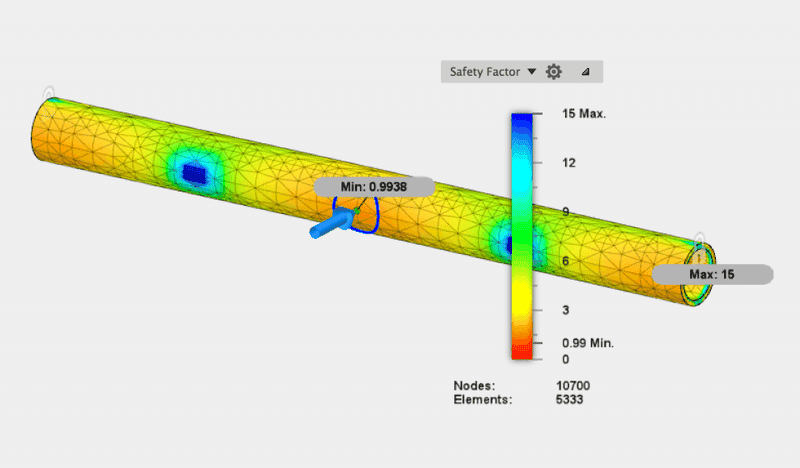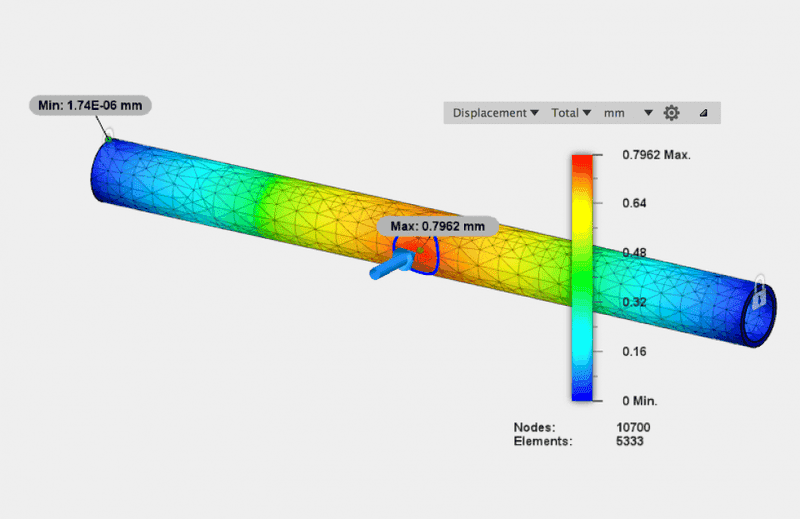10,000 N results in a minimum safety factor at the point of force of .49
6,000 N results in a minimum safety factor at the point of force of .83
5,000 N results in a minimum safety factor at the point of force of .99
4,000 N results in a minimum safety factor at the point of force of 1.2

so i figure at ~5,000 N we have a failure, and at > ~5,000 N we don't.

i also see the the FEA program shows that we have minuscule level of permanent displacement of 0.79mm.

using the formula Energy (KE) = 1/2 Force (F) * Deflection (x) i end up with:

KE = 1/2 * 5,000 * .00079 = 1.975 joules. so, that doesn't seem right.

what am i goofing up here?

Mech_Engineer
Gold Member
I would make sure and run a mesh convergence study on your FEA model before trusting its numbers. This is where you increase the density of your mesh and see if the numbers change. If they change with a higher mesh density (especially stress), your model's result is being driven by some local effects near a geometry feature, or one of your boundary conditions.

Also, your mesh looks pretty coarse; I'd recommend that you should have 2-3 elements through the thickness of the pipe's wall.

i've max'd out the elements on my FEA program, and it changed the numbers, but it didn't seem to change them much.

i'm still puzzled if maybe i've made an error in following the suggested formula, or perhaps there are limitation in my FEA program (autodesk fusion 360.)

here are shots showing the stress numbers from a fine mesh (the finest my app will let me go to) and a more coarse mesh.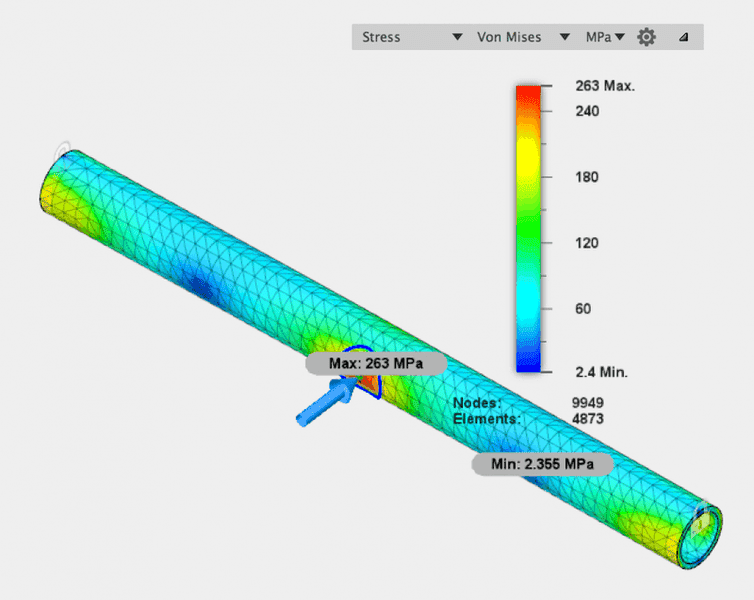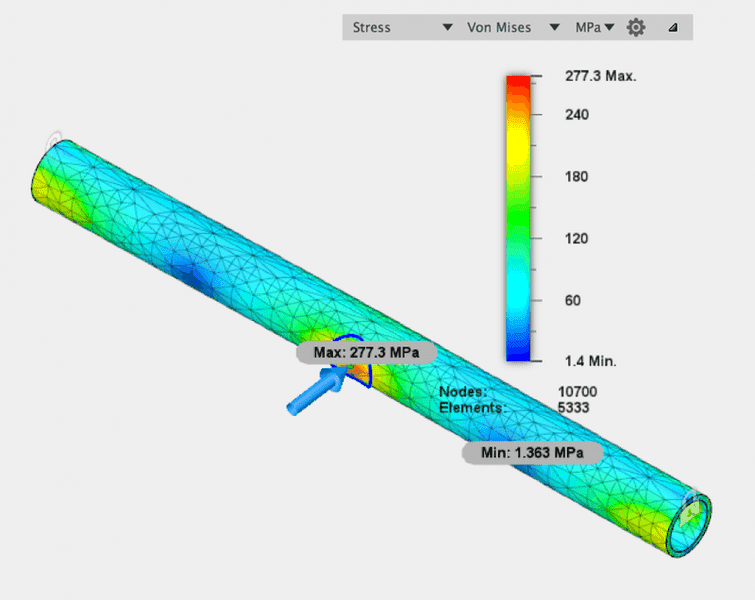Mech_Engineer
Gold Member
What are your support conditions for the pipe? Is it fixed at the ends?

Also what is the pipe's wall thickness, diameter, and length?

12" length, 1" OD, .088" wall thickness.
it's fixed at both ends.

Mech_Engineer
Gold Member
Keep in mind that fixing both ends of the pipe approximates it being welded between two infinitely stiff plates which cannot move. This results in some tension in the rod when bending. Does your analytical equation analysis take this into account?

Mech_Engineer
Gold Member
The equation provided above is for a simply supported beam, so it's not quite the same as your FEA model. If you indeed want the model to have the ends of the beam fixed, then the equation set you should use are for a statically indeterminate beam.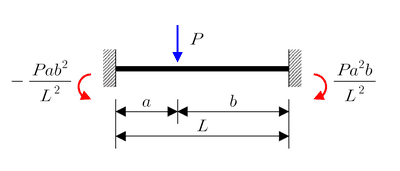jack action
Gold Member
The stiffness of your bar is 5000/0.00079 = 6.3e6 N/m

Compare with my approach. You have a single horizontal bar:

$$k_{hb} = \frac{48E_mI}{L^3}$$

But this is for a simply supported beam (as @Mech_Engineer mentioned). For the 2 ends fixed the 48 must be replaced by 192 (It is stiffer).

$E_m$ is 200e9 Pa for steel (I assumed the material), $I = \frac{\pi}{4}(r_o^4 - r_i^4)$ is 6.3e-9 m4 for the tubing and $L$ is 0.3048 m. Therefore, $k_{hb}$ is 8.5e6 N/m, i.e. only 35% stiffer than what your FEA predicted. The displacement ($x = \frac{F}{k_{hb}}$) and energy produced ($\frac{F^2}{2k_{hb}}$ or $\frac{Fx}{2}$) are then 0.59 mm and 1.46 J, which all relate to your numbers.

JBA
Gold Member
JBA,

so i gave it shot - but i must be doing something dumb. the answer isn't making a lot of sense.

here are a couple of images from my FEA program. i'm using a 12" titanium pipe, and applying force to it in a 10mm radius.

i'm using "safety factor" to determine when a failure occurs (when it < 1 the material fails, and permanently deforms.)

View attachment 101990

View attachment 101992
10,000 N results in a minimum safety factor at the point of force of .49
6,000 N results in a minimum safety factor at the point of force of .83
5,000 N results in a minimum safety factor at the point of force of .99
4,000 N results in a minimum safety factor at the point of force of 1.2

so i figure at ~5,000 N we have a failure, and at > ~5,000 N we don't.

i also see the the FEA program shows that we have minuscule level of permanent displacement of 0.79mm.

using the formula Energy (KE) = 1/2 Force (F) * Deflection (x) i end up with:

KE = 1/2 * 5,000 * .00079 = 1.975 joules. so, that doesn't seem right.

what am i goofing up here?

The ≅ 5000 Newtons for the initial failure point is correct, but you are misreading the safety factor values. As the safety factor increases to 1 and above the allowable load decreases, not the reverse. The lower than 1 safety factor value is an indication of failure and as the safety factor increases to 1 and above (and the associated load values decrease) the risk of damage to the beam decreases.